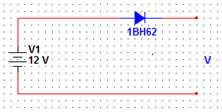Courses

# Test: The PN Junction As A Diode

## 10 Questions MCQ Test Electronic Devices | Test: The PN Junction As A Diode

Description
This mock test of Test: The PN Junction As A Diode for Electrical Engineering (EE) helps you for every Electrical Engineering (EE) entrance exam. This contains 10 Multiple Choice Questions for Electrical Engineering (EE) Test: The PN Junction As A Diode (mcq) to study with solutions a complete question bank. The solved questions answers in this Test: The PN Junction As A Diode quiz give you a good mix of easy questions and tough questions. Electrical Engineering (EE) students definitely take this Test: The PN Junction As A Diode exercise for a better result in the exam. You can find other Test: The PN Junction As A Diode extra questions, long questions & short questions for Electrical Engineering (EE) on EduRev as well by searching above.
QUESTION: 1

### How many junction/s do a diode consist?

Solution:

Diode is a one junction semiconductor device which has one cathode and anode. The junction is of p-n type.

QUESTION: 2

### If the positive terminal of the battery is connected to the anode of the diode, then it is known as

Solution:

When a positive terminal is connected to the anode, the diode is forward biased which lets the flow of the current in the circuit.

QUESTION: 3

### During reverse bias, a small current develops known as

Solution:

When the diode is reverse biased, a small current flows between the p-n junction which is of the order of the Pico ampere. This current is known as reverse saturation current.

QUESTION: 4

If the voltage of the potential barrier is V0. A voltage V is applied to the input, at what moment will the barrier disappear?

Solution:

When the voltage will be same that of the potential barrier, the potential barrier disappears resulting in flow of current.

QUESTION: 5

During the reverse biased of the diode, the back resistance decrease with the increase of the temperature. Is it true or false?

Solution:

Due to the increase in the reverse saturation current due to the increase in the temperature, the back resistance decrease with the increasing temperature.

QUESTION: 6

What is the maximum electric field when Vbi=2V , VR=5V and width of the semiconductor is 7cm?

Solution:

Emax=-2(Vbi+VR)/W
=-2(2+5)/ (7*10-2)
=-200V/m.

QUESTION: 7

When the diode is reverse biased with a voltage of 6V and Vbi=0.63V. Calculate the total potential.

Solution:

Vt=Vbi+VR
=0.63+6
=6V.

QUESTION: 8

It is possible to measure the voltage across the potential barrier through a voltmeter?

Solution:

The contacts of the voltmeter have some resistance which will not accurately measure the voltage across the potential barrier. Thus, it is not possible to measure the voltage across the potential barrier.

QUESTION: 9

What will be the output of the following circuit? (Assume 0.7V drop across the diode)​Solution:

V=12-0.7
=11.3V.

QUESTION: 10

Which of the following formula represents the correct formula for width of the depletion region?

Solution:

Option a is the correct formula.